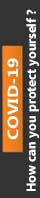We're Open

0% Plagiarism Guaranteed & Custom Written

# 1. For the circuit given in FIGURE 1 the power factor is 0.72 lagging and the power dissipated is 375 W.

MODULE TITLE : ENGINEERING SCIENCE TOPIC TITLE : A.C. CIRCUITS

MODULE LEARNING OUTCOMES Knowledge and Understanding 1. Describe and explain static engineering systems. 2. Describe and explain simple d.c.and a.c.circuits Cognitive and Intellectual Skills 3. Apply d.c. and a.c.theory to a variety of well defined circuits. Practical and Professional Skills Key Transferable Skills 4. Demonstrate numerical and statistical skills applied to engineering problems relating to electrical and mechanical science. 2 Teesside University Open Learning (Engineering) © Teesside University 2011 PASS A solution has been developed to solve problems but may include some minor redundancies or errors. MERIT Criteria in excess of the pass grade. Suitable equations identified and applied to produce correct solutions with the minimum of assistance. DISTINC

1. For the circuit given in FIGURE 1 the power factor is 0.72 lagging and the power dissipated is 375 W.

Determine the: (i) apparent power (ii) reactive power (iii) the magnitude of the current flowing in the circuit (iv) the value of the impedance Z and state whether circuit is inductive or capacitive.

2. A 50 kW load operates from a 60 Hz 10 kV rms line with a power factor of 60% lagging. Determine the capacitance that must be placed in parallel with the load to achieve a 90% lagging power factor.

3. A series RLC circuit is connected to a 5 V supply, the frequency of the supply is adjusted to give a maximum current of 11.9 mA at 2.5 kHz. The Q factor is 70. Determine the component values of the circuit

4. A single phase transformer has the following rating: 120 kVA, 2000 V/100 V, 60 Hz with 1000 primary turns. Determine: (a) the secondary turns

5. An a.c. voltage, V, comprises of a fundamental voltage of 100 V rms at a frequency of 120 Hz, a 3rd harmonic which is 20% of the fundamental, a 5th harmonic which is 10% of the fundamental and at a phase angle of 1.2 radians lagging.

(i) Write down an expression for the voltage waveform.

100% Plagiarism Free & Custom Written,International House, 12 Constance Street, London, United Kingdom,
E16 2DQ

## STILL NOT CONVINCED?

We've produced some samples of what you can expect from our Academic Writing Service - these are created by our writers to show you the kind of high-quality work you'll receive. Take a look for yourself!FLAT 25% OFF ON EVERY ORDER.Use "FLAT25" as your promo code during checkout